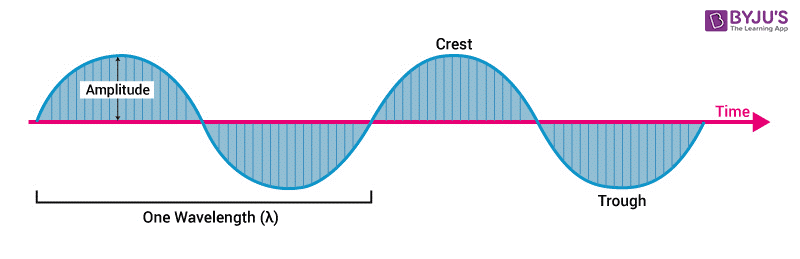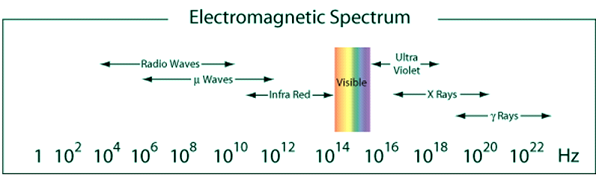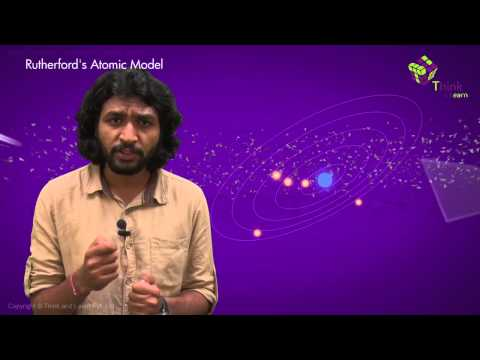Checkout JEE MAINS 2022 Question Paper Analysis : Checkout JEE MAINS 2022 Question Paper Analysis :

Initially electricity and magnetism were considered to be separate forces. Later in the year 1873, James Clerk Maxwell, the Scottish physicist, developed a combined theory known as electromagnetism. Electromagnetic field produce electromagnetic radiation also referred to as EM radiation. Electromagnetic radiation is a form of energy that is present all around us and takes various forms like microwaves, television waves, radio waves, gamma rays, X-rays, etc. In this piece of article, we will discuss electromagnetic radiation and its properties.Electromagnetic radiation can be defined as a form of energy that is produced by the movement of electrically charged particles travelling through a matter or vacuum or by oscillating magnetic and electric disturbance. The magnetic and the electric fields come at 90° to each other, and the combined waves move perpendicular to both electric and magnetic oscillating fields occurring the disturbance.## Electromagnetic Theory

Magnetism and electricity were once considered as separate forces. However, in the year 1873, Clerk Maxwell, a Scottish physicist developed a unified theory of electromagnetism. Its study deals with how the electrically charged particles interact among themselves and with the magnetic field. The main electromagnetic interactions are provided in the points mentioned below.

• Magnetic poles come in pairs that repel and attract each other, just like electric charges do.
• The force of repulsion or attraction between two electric charges is inversely proportional to the square of the distance between the particles.
• An electric field in motion produces a magnetic field.
• A wire with an electric current produces a magnetic field whose direction depends on the direction of the electric current.

When electromagnetic radiation occurs, the electron radiations are released as photons. These are bundles of light energy or quantized harmonic waves which travel at the speed of light. Then based on the wavelength of the electromagnetic spectrum, the energy is grouped into different categories. These magnetic and electric waves travel perpendicular to each other and have some characteristics like wavelength, amplitude, and frequency. Some basic properties of Electromagnetic Radiation are given in the points mentioned below.Electromagnetic Waves

• They can travel through empty space. Waves other than electromagnetic waves have to travel through some substance. For example, sound waves will need either a solid, liquid or gas to pass through.
• The speed of light which is 2.99792458 x 108 m/s is always constant.
• Wavelength is commonly characterized by the symbol ‘λ’. It is the measure between the distance of either troughs or crests.

## Electromagnetic SpectrumLight waves of various frequency in electromagnetic spectrum

Electromagnetic spectrum is the range of frequencies EM radiations along with their associated wavelengths and photon energies. It consists of Gamma-rays, X-rays, ultraviolet rays, infrared rays, radio waves, microwaves. Electromagnetic radiations have a wide range of frequencies, wavelengths and photon energy levels. These waves travel at the speed of light in vacuum.
See the video below, to have a clear idea about electromagnetism.## Waves and their Characteristics

Electromagnetic radiation occurs when an atomic particle, like an electron, is accelerated by an electric field, causing it to accelerate. Electromagnetic waves and their characteristics is explained briefly in the points mentioned below.

### Wavelength

Wavelength (λ) is the distance between successive crests of a wave, especially points in an electromagnetic wave or sound wave. It can be simply defined as the distance of one full cycle of the oscillation. If ‘λ’ is the wavelength, ‘c’ is the speed of light and ‘ν‘ is frequency. Then we can derive the relation given below.

c = λ ν

The shorter the wavelength, greater the frequency and greater the frequency, the higher the energy.

### Amplitude

It is the distance from the middle of the wave to the maximum vertical displacement of the wave.  Larger the amplitude, higher the energy and lower the amplitude, lower the energy. Amplitude tells us about the brightness or intensity of a wave compared to other waves.

### Frequency

The number of cycles per second is defined as Frequency. It is defined as Hertz (Hz) or sec-1. If ‘E’ is the energy, ‘h’ is Planck’s constant which is equal to 6.62607 x 10-34 and ‘ν‘ is the frequency we can derive the relation given below.

E = hν

Thus, we can see that frequency is directly proportional to energy.

### Period

Period is commonly characterised by the symbol ‘T’. It is the total time which a wave takes to travel 1 wavelength.

### Velocity

In relation with electromagnetic radiation, the velocity is normally expressed as:

Velocity = λν
[where, ν = frequency]

The wave velocity in vacuum for electromagnetic wave is = 186,282 miles/second or 2.99×108 m/s.

 For Better UnderstandingStay tuned with BYJU’S for more such interesting articles. Also, register to “BYJU’S – The Learning App” for loads of interactive, engaging Physics-related videos and an unlimited academic assist.

## Frequently Asked Questions – FAQs

### What is electromagnetism?

Electromagnetism is a branch of physics that deals with the study of electromagnetic forces. Interaction between electrically charged particles and fields associated with them is known as electromagnetic forces.

True.

### Define wavelength.

Wavelength (λ) is the distance between successive crests of a wave, especially points in an electromagnetic wave or sound wave.

### What is the velocity of electromagnetic waves in vacuum?

Velocity of electromagnetic waves in vacuum is 186,282 miles/second.

Electromagnetic radiation occurs when an atomic particle like an electron, is accelerated by an electric field, causing it to accelerate.